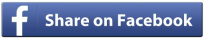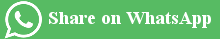Body Surface Area Calculator (BSA Calculation Formula)Body Surface Area (BSA) is a calculation of surface area of human body by using his/her height and weight. It is expressed in square meters (m2). It is used by physicians to calculate to dosage of some important drugs according to your body.

BSA Calculation Formula
BSA = Sqrt (Body Weight (kg) x Height (cm) / 3600)

Here Sqrt means square root. Body weight should be provided in kg and height in cm. (However you may provide it in any unit you want in the calculator provided below). The formula given above is also known as Mosteller Method to calculate BSA. There are some other methods as well but its the most widely used one.

Body Surface Area Calculator / BSA Calculator

BSA Calculator / Body Surface Area Calculator

 Height: 1'2'3'4'5'6'7'1" 2"3"4"5"6"7"8"9"10"11" Cms : Weight: KgPounds
 BSA (Body Surface Area)(m²):

Average BSA value for different age and gender
 Neonate (newborn) 0.25 m² Child of 2 years 0.5 m² 9 years 1.07 m² 10 years 1.14 m² 12–13 years 1.33 m² Women 1.6 m² Men 1.9 m²

Usage of Body Surface Area in different treatments

• To determine the dosage of medicine according to the body of patient.
• It saves from the risk of overdosing or underdosing.
• Chemotherapy dosage is done according to patient's BSA